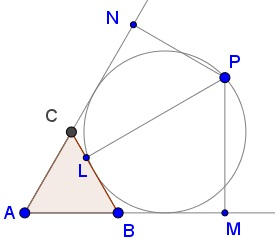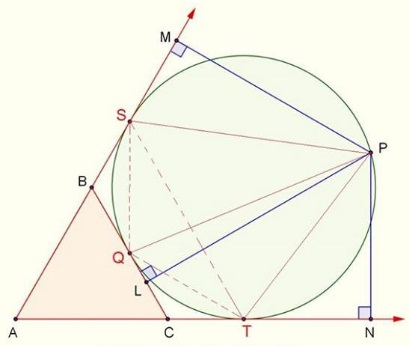# Excircle in Equilateral Triangle

### Source### Problem

Point $P\,$ is on the excircle $(O_a)\,$ of $\Delta ABC.\,$ Points $M,N,P\,$ are the projections of $P\,$ on $BC\,$ and on the extensions of $AB\,$ and $AC,\,$ respectively.Find

$\displaystyle E=\frac{\sqrt{MP}+\sqrt{NP}}{\sqrt{LP}}.$

### Solution 1

Choose the circle $x^2+y^2=1.\,$ Since that's the $A\text{-excircle}\,$ of $\Delta ABC,\,$ we'll choose $BC:\,x\sqrt{3}+y=2,\,$ $AC:\,x\sqrt{3}-y+2,\,$ $AB:\,y=-1,\,$ and $P=(\cos t,\sin t),\,$ with $\displaystyle -\frac{\pi}{2}\lt t\lt\frac{5\pi}{6}.$We get $MP=1+\sin t=1+\cos \left(\frac{\pi}{2}-t\right)=2\cos^2\left(\frac{\pi}{4}-\frac{t}{2}\right),\,$ implying $\sqrt{MP}=\sqrt{2}\displaystyle\cos\left(\frac{\pi}{4}-\frac{t}{2}\right).\,$ Similarly,

$\displaystyle NP=\left|\frac{\sqrt{3}}{2}\cos t-\frac{1}{2}\sin t+1\right|=1+\cos\left(\frac{\pi}{6}+t\right)=2\cos^2\left(\frac{\pi}{12}+\frac{t}{2}\right),$

implying $\displaystyle\sqrt{NP}=\sqrt{2}\cos\left(\frac{\pi}{12}+\frac{t}{2}\right).\,$ Further,

$\displaystyle LP=\left|\frac{\sqrt{3}}{2}\cos t+\frac{1}{2}\sin t+1\right|=1+\cos\left(\frac{\pi}{6}-t\right)=2\cos^2\left(\frac{\pi}{12}-\frac{t}{2}\right),$

implying $\displaystyle\sqrt{LP}=\sqrt{2}\cos\left(\frac{\pi}{12}-\frac{t}{2}\right).\,$ From here,

$\displaystyle\frac{\sqrt{MP}+\sqrt{NP}}{\sqrt{LP}}=\frac{\displaystyle\cos\left(\frac{\pi}{12}+\frac{t}{2}\right)+\cos\left(\frac{\pi}{4}-\frac{t}{2}\right)}{\displaystyle\cos\left(\frac{\pi}{12}-\frac{t}{2}\right)}=\frac{\sqrt{3}\displaystyle\cos\left(\frac{\pi}{12}-\frac{t}{2}\right)}{\displaystyle\cos\left(\frac{\pi}{12}-\frac{t}{2}\right)}=\sqrt{3}.$

### Solution 2

In what follows we'll invoke repeatedly the following result from the diagram below.Assuming $R\,$ is the radius of the excircle, we apply the above lemma to three triangles below:$PS=\sqrt{MP\cdot(2R)},\,$ $PT=\sqrt{NP\cdot(2R)},\,$ $PQ=\sqrt{LP\cdot(2R)}.\,$

$\overparen{SQ}=\overparen{QT}=60^{\circ},\,$ hence, $\overparen{ST}=120^{\circ},\,$ so that $QS=QT=R\,$ and, subsequently, $ST=R\sqrt{3}.$

By Ptolemy's theorem in quadrilateral $QSPT,\,$ $PQ\cdot ST=PT\cdot QS+PS\cdot QT.\,$ A substitution then gives

$\sqrt{LP\cdot (2R)}\cdot R\sqrt{3}=\sqrt{NP\cdot (2R)}\cdot R+\sqrt{MP\cdot ((2R)}\cdot R,$

i.e., $\sqrt{LP}\sqrt{3}=\sqrt{MP}+\sqrt{NP}.$

### Acknowledgment

Leo Giugiuc has kindly communicated to me another problem from the peru geometrico facebook group. The problem has been posted by Edson Curahua Ortega, with credits to Edson Curahua. Solution 1 is by Leo Giugiuc; Solution 2 is by John Ascona Briceño.

• Angle Trisectors on Circumcircle
• Equilateral Triangles On Sides of a Parallelogram
• Pompeiu's Theorem
• Pairs of Areas in Equilateral Triangle
• The Eutrigon Theorem
• Equilateral Triangle in Equilateral Triangle
• Seven Problems in Equilateral Triangle
• Spiral Similarity Leads to Equilateral Triangle
• Parallelogram and Four Equilateral Triangles
• A Pedal Property in Equilateral Triangle
• Miguel Ochoa's van Schooten Like Theorem
• Two Conditions for a Triangle to Be Equilateral
• Incircle in Equilateral Triangle
• When Is Triangle Equilateral: Marian Dinca's Criterion
• Barycenter of Cevian Triangle
• Converse Construction in Pompeiu's Theorem
• Wonderful Trigonometry In Equilateral Triangle
• 60o Angle And Importance of Being The Other End of a Diameter
• One More Property of Equilateral Triangles
• Van Khea's Quickie
• Equilateral Triangle from Three Centroids
•